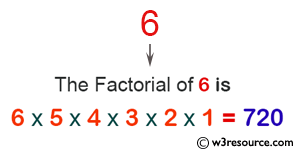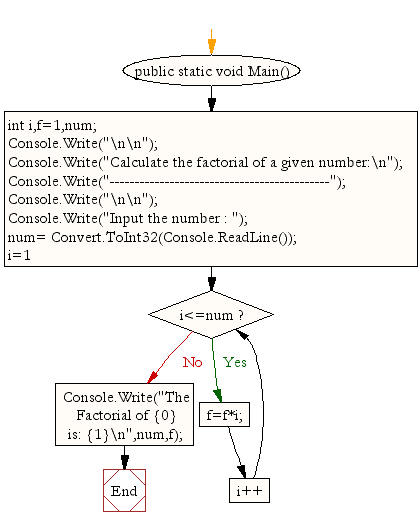﻿ C# - Calculate the factorial of a given number - w3resource# C# Sharp Exercises: Calculate the factorial of a given number

## C# Sharp For Loop: Exercise-15 with Solution

Write a C# Sharp program to calculate the factorial of a given number.

Pictorial Presentation:Sample Solution:

C# Sharp Code:

``````using System;
public class Exercise15
{
public static void Main()
{
int i,f=1,num;

Console.Write("\n\n");
Console.Write("Calculate the factorial of a given number:\n");
Console.Write("--------------------------------------------");
Console.Write("\n\n");

Console.Write("Input the number : ");
for(i=1;i<=num;i++)
f=f*i;

Console.Write("The Factorial of {0} is: {1}\n",num,f);
}
}
```
```

Sample Output:

```Calculate the factorial of a given number:
--------------------------------------------
Input the number : 6
The Factorial of 6 is: 720
```

Flowchart:C# Sharp Code Editor:

Contribute your code and comments through Disqus.

What is the difficulty level of this exercise?

Test your Programming skills with w3resource's quiz.

﻿